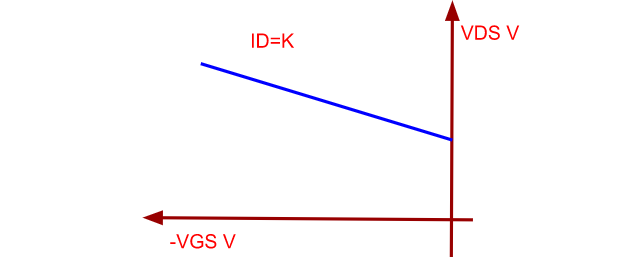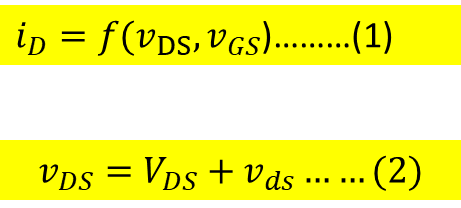### Amplification Factor 𝝁

Amplification Factor 𝝁

Another parameter of importance is called the Amplification factor and is represented by the symbol 𝝁

This is obtained from the plot drawn between the VDS and VGS(negative value) keeping the value of the Drain current ID constant.Figure 10: Amplification Characteristic

The Amplification factor  𝝁 represents the maximum amount of amplification the JFET can produce.

Relationship between the Amplification Factor 𝝁, Transconductance gm and Drain Resistance rd:From equation (1), it may be noted that iD is the instantaneous value of the Drain current, vDS is the instantaneous value of Drain - Source Voltage and vGS is the instantaneous value of the Gate - Source voltage.

It may be noted here that the word instantaneous value represents the variable DC value.

It may also be observed from the equation (1) that the total variation in the value of the Drain current iD is the sum of the variations in vDS and vGS

Ilustration:

For this look at the equation (2).

Here

vDS  → Variable DC

VDS → Pure DC

vds   → Pure AC

Expand the equation (1) using Taylor's expansion, we getFrom (3) note the following:

vDS → Small change in vDS

vGS → Small change in vGS

iD → The corresponding change in iD